# Potentials in galpy¶

galpy contains a large variety of potentials in galpy.potential that can be used for orbit integration, the calculation of action-angle coordinates, as part of steady-state distribution functions, and to study the properties of gravitational potentials. This section introduces some of these features.

## Potentials and forces¶

Various 3D and 2D potentials are contained in galpy, list in the API page. Another way to list the latest overview of potentials included with galpy is to run

>>> import galpy.potential
>>> print([p for p in dir(galpy.potential) if 'Potential' in p])
# ['CosmphiDiskPotential',
#  'DehnenBarPotential',
#  'DoubleExponentialDiskPotential',
#  'EllipticalDiskPotential',
#  'FlattenedPowerPotential',
#  'HernquistPotential',
# ....]


(list cut here for brevity). Section Rotation curves explains how to initialize potentials and how to display the rotation curve of single Potential instances or of combinations of such instances. Similarly, we can evaluate a Potential instance

>>> from galpy.potential import MiyamotoNagaiPotential
>>> mp= MiyamotoNagaiPotential(a=0.5,b=0.0375,normalize=1.)
>>> mp(1.,0.)
# -1.2889062500000001


Most member functions of Potential instances have corresponding functions in the galpy.potential module that allow them to be evaluated for lists of multiple Potential instances (and in versions >=1.4 even for nested lists of Potential instances). galpy.potential.MWPotential2014 is such a list of three Potential instances

>>> from galpy.potential import MWPotential2014
>>> print(MWPotential2014)
# [<galpy.potential.PowerSphericalPotentialwCutoff.PowerSphericalPotentialwCutoff instance at 0x1089b23b0>, <galpy.potential.MiyamotoNagaiPotential.MiyamotoNagaiPotential instance at 0x1089b2320>, <galpy.potential.TwoPowerSphericalPotential.NFWPotential instance at 0x1089b2248>]


and we can evaluate the potential by using the evaluatePotentials function

>>> from galpy.potential import evaluatePotentials
>>> evaluatePotentials(MWPotential2014,1.,0.)
# -1.3733506513947895


Tip

Lists of Potential instances can be nested, allowing you to easily add components to existing gravitational-potential models. For example, to add a DehnenBarPotential to MWPotential2014, you can do: pot= [MWPotential2014,DehnenBarPotential()] and then use this pot everywhere where you can use a list of Potential instances. You can also add potential simply as pot= MWPotential2014+DehnenBarPotential().

Warning

galpy potentials do not necessarily approach zero at infinity. To compute, for example, the escape velocity or whether or not an orbit is unbound, you need to take into account the value of the potential at infinity. E.g., $$v_{\mathrm{esc}}(r) = \sqrt{2[\Phi(\infty)-\Phi(r)]}$$.

Tip

As discussed in the section on physical units, potentials can be initialized and evaluated with arguments specified as a astropy Quantity with units. Use the configuration parameter apy-units = True to get output values as a Quantity. See also the subsection on Initializing potentials with parameters with units below.

We can plot the potential of axisymmetric potentials (or of non-axisymmetric potentials at phi=0) using the plot member function

>>> mp.plot()


which produces the following plot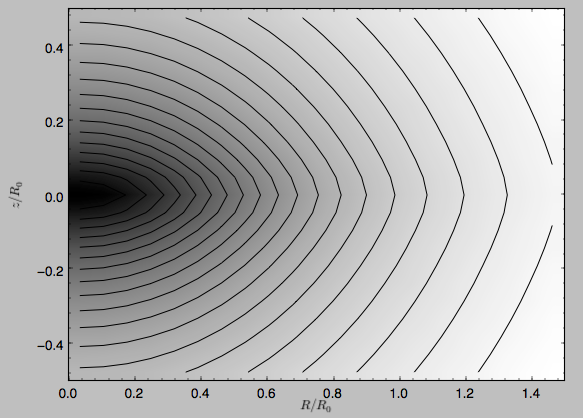Similarly, we can plot combinations of Potentials using plotPotentials, e.g.,

>>> from galpy.potential import plotPotentials
>>> plotPotentials(MWPotential2014,rmin=0.01)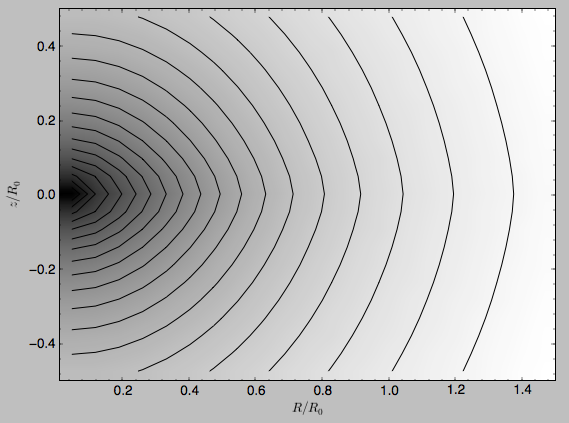These functions have arguments that can provide custom R and z ranges for the plot, the number of grid points, the number of contours, and many other parameters determining the appearance of these figures.

galpy also allows the forces corresponding to a gravitational potential to be calculated. Again for the Miyamoto-Nagai Potential instance from above

>>> mp.Rforce(1.,0.)
# -1.0


This value of -1.0 is due to the normalization of the potential such that the circular velocity is 1. at R=1. Similarly, the vertical force is zero in the mid-plane

>>> mp.zforce(1.,0.)
# -0.0


but not further from the mid-plane

>>> mp.zforce(1.,0.125)
# -0.53488743705310848


As explained in Units in galpy, these forces are in standard galpy units, and we can convert them to physical units using methods in the galpy.util.bovy_conversion module. For example, assuming a physical circular velocity of 220 km/s at R=8 kpc

>>> from galpy.util import bovy_conversion
>>> mp.zforce(1.,0.125)*bovy_conversion.force_in_kmsMyr(220.,8.)
# -3.3095671288657584 #km/s/Myr
>>> mp.zforce(1.,0.125)*bovy_conversion.force_in_2piGmsolpc2(220.,8.)
# -119.72021771473301 #2 \pi G Msol / pc^2


Again, there are functions in galpy.potential that allow for the evaluation of the forces for lists of Potential instances, such that

>>> from galpy.potential import evaluateRforces
>>> evaluateRforces(MWPotential2014,1.,0.)
# -1.0
>>> from galpy.potential import evaluatezforces
>>> evaluatezforces(MWPotential2014,1.,0.125)*bovy_conversion.force_in_2piGmsolpc2(220.,8.)
>>> -69.680720137571114 #2 \pi G Msol / pc^2


We can evaluate the flattening of the potential as $$\sqrt{|z\,F_R/R\,F_Z|}$$ for a Potential instance as well as for a list of such instances

>>> mp.flattening(1.,0.125)
# 0.4549542914935209
>>> from galpy.potential import flattening
>>> flattening(MWPotential2014,1.,0.125)
# 0.61231675305658628


## Densities¶

galpy can also calculate the densities corresponding to gravitational potentials. For many potentials, the densities are explicitly implemented, but if they are not, the density is calculated using the Poisson equation (second derivatives of the potential have to be implemented for this). For example, for the Miyamoto-Nagai potential, the density is explicitly implemented

>>> mp.dens(1.,0.)
# 1.1145444383277576


and we can also calculate this using the Poisson equation

>>> mp.dens(1.,0.,forcepoisson=True)
# 1.1145444383277574


which are the same to machine precision

>>> mp.dens(1.,0.,forcepoisson=True)-mp.dens(1.,0.)
# -2.2204460492503131e-16


Similarly, all of the potentials in galpy.potential.MWPotential2014 have explicitly-implemented densities, so we can do

>>> from galpy.potential import evaluateDensities
>>> evaluateDensities(MWPotential2014,1.,0.)
# 0.57508603122264867


In physical coordinates, this becomes

>>> evaluateDensities(MWPotential2014,1.,0.)*bovy_conversion.dens_in_msolpc3(220.,8.)
# 0.1010945632524705 #Msol / pc^3


We can also plot densities

>>> from galpy.potential import plotDensities
>>> plotDensities(MWPotential2014,rmin=0.1,zmax=0.25,zmin=-0.25,nrs=101,nzs=101)


which gives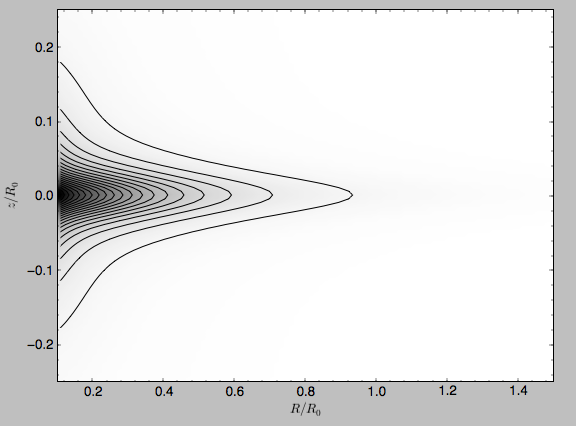Another example of this is for an exponential disk potential

>>> from galpy.potential import DoubleExponentialDiskPotential
>>> dp= DoubleExponentialDiskPotential(hr=1./4.,hz=1./20.,normalize=1.)


The density computed using the Poisson equation now requires multiple numerical integrations, so the agreement between the analytical density and that computed using the Poisson equation is slightly less good, but still better than a percent

>>> (dp.dens(1.,0.,forcepoisson=True)-dp.dens(1.,0.))/dp.dens(1.,0.)
# 0.0032522956769123019


The density is

>>> dp.plotDensity(rmin=0.1,zmax=0.25,zmin=-0.25,nrs=101,nzs=101)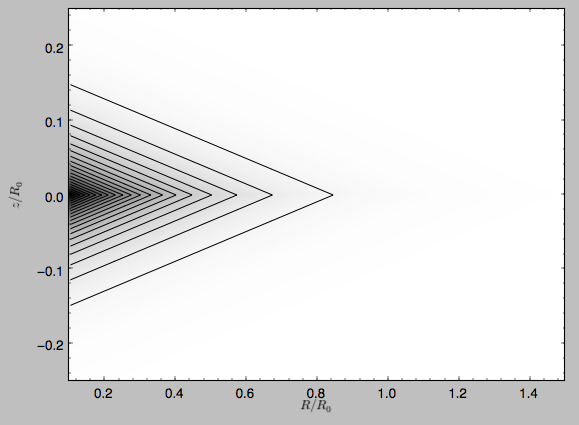and the potential is

>>> dp.plot(rmin=0.1,zmin=-0.25,zmax=0.25)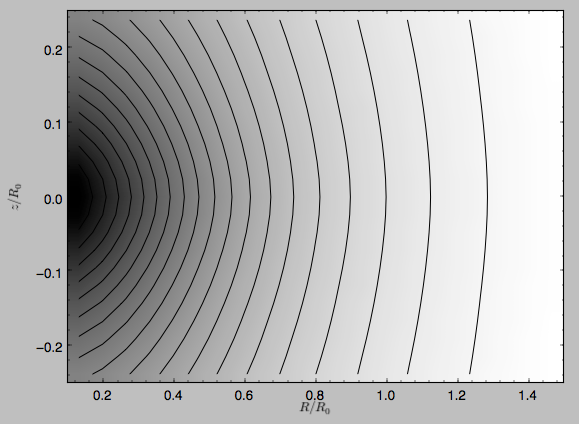Clearly, the potential is much less flattened than the density.

## Modifying potential instances using wrappers¶

Potentials implemented in galpy can be modified using different kinds of wrappers. These wrappers modify potentials to, for example, change their amplitude as a function of time (e.g., to grow or decay the bar contribution to a potential) or to make a potential rotate. Specific kinds of wrappers are listed on the Potential wrapper API page. These wrappers can be applied to instances of any potential implemented in galpy (including other wrappers). An example is to grow a bar using the polynomial smoothing of Dehnen (2000). We first setup an instance of a DehnenBarPotential that is essentially fully grown already

>>> from galpy.potential import DehnenBarPotential
>>> dpn= DehnenBarPotential(tform=-100.,tsteady=0.) # DehnenBarPotential has a custom implementation of growth that we ignore by setting tform to -100


and then wrap it

>>> from galpy.potential import DehnenSmoothWrapperPotential


This grows the DehnenBarPotential starting at 4 bar periods before t=0 over a period of 2 bar periods. DehnenBarPotential has an older, custom implementation of the same smoothing and the (tform,tsteady) pair used here corresponds to the default setting for DehnenBarPotential. Thus we can compare the two

>>> dp= DehnenBarPotential()
>>> print(dp(0.9,0.3,phi=3.,t=-2.)-dswp(0.9,0.3,phi=3.,t=-2.))
# 0.0
>>> print(dp.Rforce(0.9,0.3,phi=3.,t=-2.)-dswp.Rforce(0.9,0.3,phi=3.,t=-2.))
# 0.0


The wrapper SolidBodyRotationWrapperPotential allows one to make any potential rotate around the z axis. This can be used, for example, to make general bar-shaped potentials, which one could construct from a basis-function expansion with SCFPotential, rotate without having to implement the rotation directly. As an example consider this SoftenedNeedleBarPotential (which has a potential-specific implementation of rotation)

>>> sp= SoftenedNeedleBarPotential(normalize=1.,omegab=1.8,pa=0.)


The same potential can be obtained from a non-rotating SoftenedNeedleBarPotential run through the SolidBodyRotationWrapperPotential to add rotation

>>> sp_still= SoftenedNeedleBarPotential(omegab=0.,pa=0.,normalize=1.)
>>> swp= SolidBodyRotationWrapperPotential(pot=sp_still,omega=1.8,pa=0.)


Compare for example

>>> print(sp(0.8,0.2,phi=0.2,t=3.)-swp(0.8,0.2,phi=0.2,t=3.))
# 0.0
>>> print(sp.Rforce(0.8,0.2,phi=0.2,t=3.)-swp.Rforce(0.8,0.2,phi=0.2,t=3.))
# 8.881784197e-16


Wrapper potentials can be used anywhere in galpy where general potentials can be used. They can be part of lists of Potential instances. They can also be used in C for orbit integration provided that both the wrapper and the potentials that it wraps are implemented in C. For example, a static LogarithmicHaloPotential with a bar potential grown as above would be

>>> from galpy.potential import LogarithmicHaloPotential, evaluateRforces
>>> lp= LogarithmicHaloPotential(normalize=1.)
>>> pot= lp+dswp
>>> print(evaluateRforces(pot,0.9,0.3,phi=3.,t=-2.))
# -1.00965326579


Tip

To simply adjust the amplitude of a Potential instance, you can multiply the instance with a number or divide it by a number. For example, pot= 2.*LogarithmicHaloPotential(amp=1.) is equivalent to pot= LogarithmicHaloPotential(amp=2.). This is useful if you want to, for instance, quickly adjust the mass of a potential.

## Close-to-circular orbits and orbital frequencies¶

We can also compute the properties of close-to-circular orbits. First of all, we can calculate the circular velocity and its derivative

>>> mp.vcirc(1.)
# 1.0
>>> mp.dvcircdR(1.)
# -0.163777427566978


or, for lists of Potential instances

>>> from galpy.potential import vcirc
>>> vcirc(MWPotential2014,1.)
# 1.0
>>> from galpy.potential import dvcircdR
>>> dvcircdR(MWPotential2014,1.)
# -0.10091361254334696


We can also calculate the various frequencies for close-to-circular orbits. For example, the rotational frequency

>>> mp.omegac(0.8)
# 1.2784598203204887
>>> from galpy.potential import omegac
>>> omegac(MWPotential2014,0.8)
# 1.2733514576122869


and the epicycle frequency

>>> mp.epifreq(0.8)
# 1.7774973530267848
>>> from galpy.potential import epifreq
>>> epifreq(MWPotential2014,0.8)
# 1.7452189766287691


as well as the vertical frequency

>>> mp.verticalfreq(1.0)
# 3.7859388972001828
>>> from galpy.potential import verticalfreq
>>> verticalfreq(MWPotential2014,1.)
# 2.7255405754769875


We can also for example easily make the diagram of $$\Omega-n \kappa /m$$ that is important for understanding kinematic spiral density waves. For example, for MWPotential2014

>>> def OmegaMinusKappa(pot,Rs,n,m,ro=8.,vo=220.): # ro,vo for physical units
return omegac(pot,Rs,ro=ro,vo=vo)-n/m*epifreq(pot,Rs,ro=ro,vo=vo)
>>> plot(Rs,OmegaMinusKappa(MWPotential2014,Rs,0,1))
>>> plot(Rs,OmegaMinusKappa(MWPotential2014,Rs,1,2))
>>> plot(Rs,OmegaMinusKappa(MWPotential2014,Rs,1,1))
>>> plot(Rs,OmegaMinusKappa(MWPotential2014,Rs,1,-2))
>>> ylim(-20.,100.)
>>> xlabel(r'$R\,(\mathrm{kpc})$')
>>> ylabel(r'$(\mathrm{km\,s}^{-1}\,\mathrm{kpc}^{-1})$')
>>> text(3.,21.,r'$\Omega-\kappa/2$',size=18.)
>>> text(5.,50.,r'$\Omega$',size=18.)
>>> text(7.,60.,r'$\Omega+\kappa/2$',size=18.)
>>> text(6.,-7.,r'$\Omega-\kappa$',size=18.)


which gives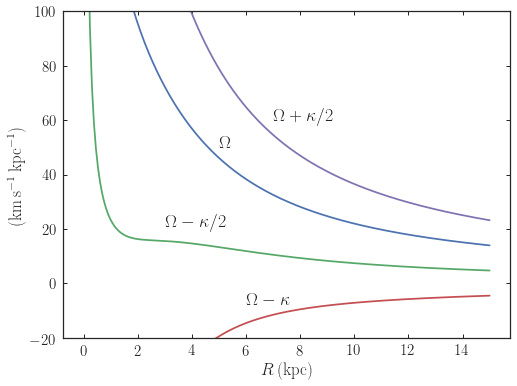For close-to-circular orbits, we can also compute the radii of the Lindblad resonances. For example, for a frequency similar to that of the Milky Way’s bar

>>> mp.lindbladR(5./3.,m='corotation') #args are pattern speed and m of pattern
# 0.6027911166042229 #~ 5kpc
# None
# 0.9906190683480501


The None here means that there is no inner Lindblad resonance, the m=-2 resonance is in the Solar neighborhood (see the section on the Hercules stream in this documentation).

## Using interpolations of potentials¶

galpy contains a general Potential class interpRZPotential that can be used to generate interpolations of potentials that can be used in their stead to speed up calculations when the calculation of the original potential is computationally expensive (for example, for the DoubleExponentialDiskPotential). Full details on how to set this up are given here. Interpolated potentials can be used anywhere that general three-dimensional galpy potentials can be used. Some care must be taken with outside-the-interpolation-grid evaluations for functions that use C to speed up computations.

## Initializing potentials with parameters with units¶

As already discussed in the section on physical units, potentials in galpy can be specified with parameters with units since v1.2. For most inputs to the initialization it is straightforward to know what type of units the input Quantity needs to have. For example, the scale length parameter a= of a Miyamoto-Nagai disk needs to have units of distance.

The amplitude of a potential is specified through the amp= initialization parameter. The units of this parameter vary from potential to potential. For example, for a logarithmic potential the units are velocity squared, while for a Miyamoto-Nagai potential they are units of mass. Check the documentation of each potential on the API page for the units of the amp= parameter of the potential that you are trying to initialize and please report an Issue if you find any problems with this.

## General density/potential pairs with basis-function expansions¶

galpy allows for the potential and forces of general, time-independent density functions to be computed by expanding the potential and density in terms of basis functions. This is supported for ellipsoidal-ish as well as for disk-y density distributions, in both cases using the basis-function expansion of the self-consistent-field (SCF) method of Hernquist & Ostriker (1992). On its own, the SCF technique works well for ellipsoidal-ish density distributions, but using a trick due to Kuijken & Dubinski (1995) it can also be made to work well for disky potentials. We first describe the basic SCF implementation and then discuss how to use it for disky potentials.

The basis-function approach in the SCF method is implemented in the SCFPotential class, which is also implemented in C for fast orbit integration. The coefficients of the basis-function expansion can be computed using the scf_compute_coeffs_spherical (for spherically-symmetric density distribution), scf_compute_coeffs_axi (for axisymmetric densities), and scf_compute_coeffs (for the general case). The coefficients obtained from these functions can be directly fed into the SCFPotential initialization. The basis-function expansion has a free scale parameter a, which can be specified for the scf_compute_coeffs_XX functions and for the SCFPotential itself. Make sure that you use the same a! Note that the general functions are quite slow.

The simplest example is that of the Hernquist potential, which is the lowest-order basis function. When we compute the first ten radial coefficients for this density we obtain that only the lowest-order coefficient is non-zero

>>> from galpy.potential import HernquistPotential
>>> from galpy.potential import scf_compute_coeffs_spherical
>>> hp= HernquistPotential(amp=1.,a=2.)
>>> Acos, Asin= scf_compute_coeffs_spherical(hp.dens,10,a=2.)
>>> print(Acos)
# array([[[  1.00000000e+00]],
#         [[ -2.83370393e-17]],
#         [[  3.31150709e-19]],
#         [[ -6.66748299e-18]],
#         [[  8.19285777e-18]],
#         [[ -4.26730651e-19]],
#         [[ -7.16849567e-19]],
#         [[  1.52355608e-18]],
#         [[ -2.24030288e-18]],
#         [[ -5.24936820e-19]]])


As a more complicated example, consider a prolate NFW potential

>>> from galpy.potential import TriaxialNFWPotential
>>> np= TriaxialNFWPotential(normalize=1.,c=1.4,a=1.)


and we compute the coefficients using the axisymmetric scf_compute_coeffs_axi

>>> a_SCF= 50. # much larger a than true scale radius works well for NFW
>>> Acos, Asin= scf_compute_coeffs_axi(np.dens,80,40,a=a_SCF)
>>> sp= SCFPotential(Acos=Acos,Asin=Asin,a=a_SCF)


If we compare the densities along the R=Z line as

>>> xs= numpy.linspace(0.,3.,1001)
>>> loglog(xs,np.dens(xs,xs))
>>> loglog(xs,sp.dens(xs,xs))


we get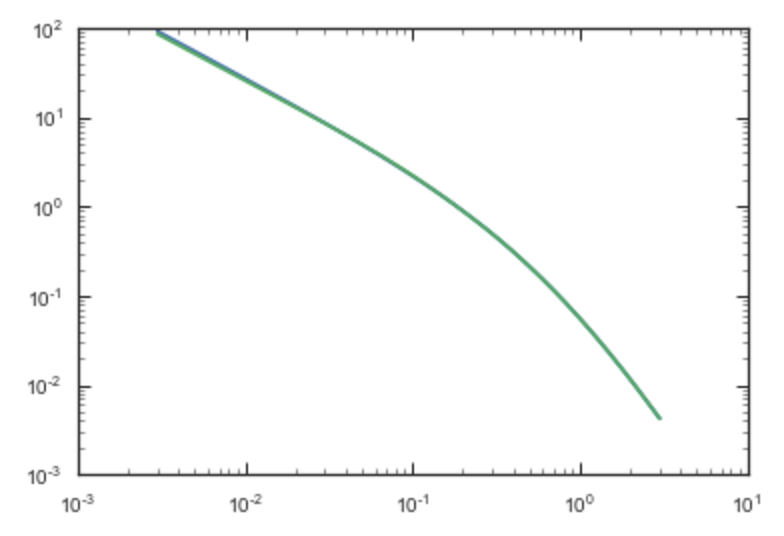If we then integrate an orbit, we also get good agreement

>>> from galpy.orbit import Orbit
>>> o= Orbit([1.,0.1,1.1,0.1,0.3,0.])
>>> ts= numpy.linspace(0.,100.,10001)
>>> o.integrate(ts,hp)
>>> o.plot()
>>> o.integrate(ts,sp)
>>> o.plot(overplot=True)


which gives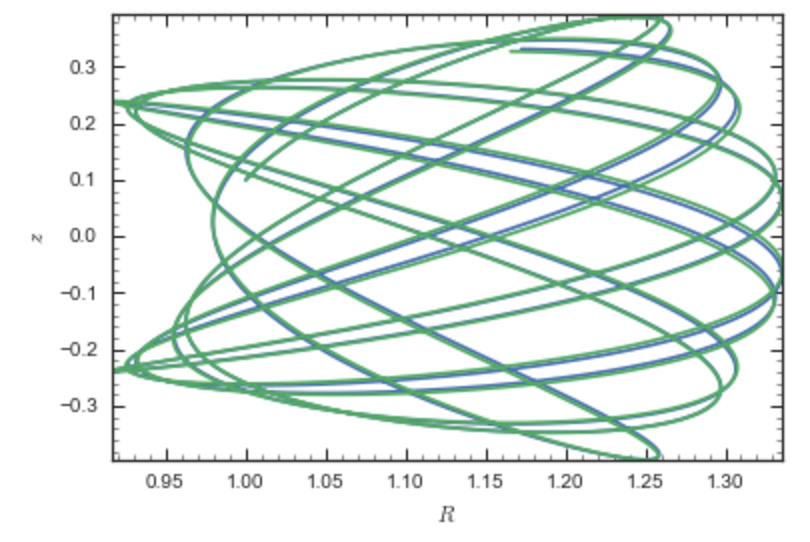Near the end of the orbit integration, the slight differences between the original potential and the basis-expansion version cause the two orbits to deviate from each other.

To use the SCF method for disky potentials, we use the trick from Kuijken & Dubinski (1995). This trick works by approximating the disk density as $$\rho_{\mathrm{disk}}(R,\phi,z) \approx \sum_i \Sigma_i(R)\,h_i(z)$$, with $$h_i(z) = \mathrm{d}^2 H(z) / \mathrm{d} z^2$$ and searching for solutions of the form

$\Phi(R,\phi,z = \Phi_{\mathrm{ME}}(R,\phi,z) + 4\pi G\sum_i \Sigma_i(r)\,H_i(z)\,,$

where $$r$$ is the spherical radius $$r^2 = R^2+z^2$$. The density which gives rise to $$\Phi_{\mathrm{ME}}(R,\phi,z)$$ is not strongly confined to a plane when $$\rho_{\mathrm{disk}}(R,\phi,z) \approx \sum_i \Sigma_i(R)\,h_i(z)$$ and can be obtained using the SCF basis-function-expansion technique discussed above. See the documentation of the DiskSCFPotential class for more details on this procedure.

As an example, consider a double-exponential disk, which we can compare to the DoubleExponentialDiskPotential implementation

>>> from galpy import potential
>>> dp= potential.DoubleExponentialDiskPotential(amp=13.5,hr=1./3.,hz=1./27.)


and then setup the DiskSCFPotential approximation to this as

>>> dscfp= potential.DiskSCFPotential(dens=lambda R,z: dp.dens(R,z),
Sigma={'type':'exp','h':1./3.,'amp':1.},
hz={'type':'exp','h':1./27.},
a=1.,N=10,L=10)


The dens= keyword specifies the target density, while the Sigma= and hz= inputs specify the approximation functions $$\Sigma_i(R)$$ and $$h_i(z)$$. These are specified as dictionaries here for a few pre-defined approximation functions, but general functions are supported as well. Care should be taken that the dens= input density and the approximation functions have the same normalization. We can compare the density along the R=10 z line as

>>> xs= numpy.linspace(0.3,2.,1001)
>>> semilogy(xs,dp.dens(xs,xs/10.))
>>> semilogy(xs,dscfp.dens(xs,xs/10.))


which gives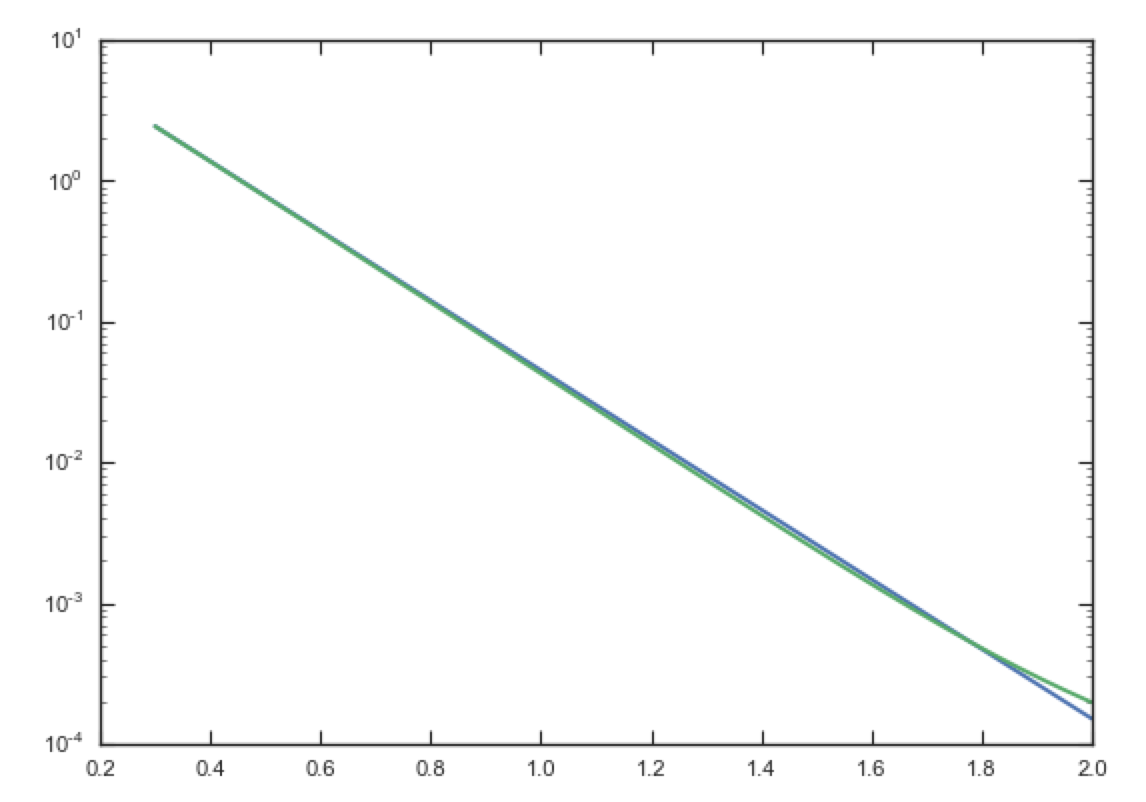The agreement is good out to 5 scale lengths and scale heights and then starts to degrade. We can also integrate orbits and compare them

>>> from galpy.orbit import Orbit
>>> o= Orbit([1.,0.1,0.9,0.,0.1,0.])
>>> ts= numpy.linspace(0.,100.,10001)
>>> o.integrate(ts,dp)
>>> o.plot()
>>> o.integrate(ts,dscfp)
>>> o.plot(overplot=True)


which gives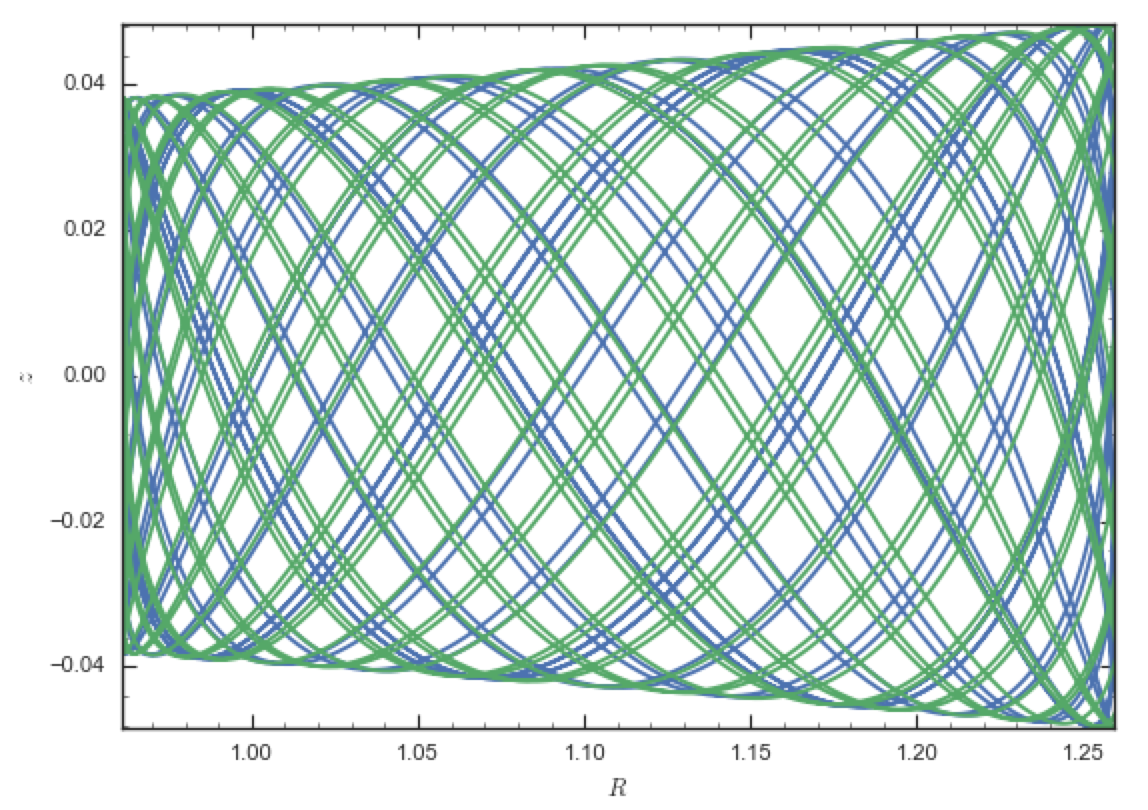The orbits diverge slightly because the potentials are not quite the same, but have very similar properties otherwise (peri- and apogalacticons, eccentricity, …). By increasing the order of the SCF approximation, the potential can be gotten closer to the target density. Note that orbit integration in the DiskSCFPotential is much faster than that of the DoubleExponentialDisk potential

>>> timeit(o.integrate(ts,dp))
# 1 loops, best of 3: 5.83 s per loop
>>> timeit(o.integrate(ts,dscfp))
# 1 loops, best of 3: 286 ms per loop


The SCFPotential and DiskSCFPotential can be used wherever general potentials can be used in galpy.

## The potential of N-body simulations¶

galpy can setup and work with the frozen potential of an N-body simulation. This allows us to study the properties of such potentials in the same way as other potentials in galpy. We can also investigate the properties of orbits in these potentials and calculate action-angle coordinates, using the galpy framework. Currently, this functionality is limited to axisymmetrized versions of the N-body snapshots, although this capability could be somewhat straightforwardly expanded to full triaxial potentials. The use of this functionality requires pynbody to be installed; the potential of any snapshot that can be loaded with pynbody can be used within galpy.

As a first, simple example of this we look at the potential of a single simulation particle, which should correspond to galpy’s KeplerPotential. We can create such a single-particle snapshot using pynbody by doing

>>> import pynbody
>>> s= pynbody.new(star=1)
>>> s['mass']= 1.
>>> s['eps']= 0.


and we get the potential of this snapshot in galpy by doing

>>> from galpy.potential import SnapshotRZPotential


With these definitions, this snapshot potential should be the same as KeplerPotential with an amplitude of one, which we can test as follows

>>> from galpy.potential import KeplerPotential
>>> kp= KeplerPotential(amp=1.)
>>> print(sp(1.1,0.),kp(1.1,0.),sp(1.1,0.)-kp(1.1,0.))
# (-0.90909090909090906, -0.9090909090909091, 0.0)
>>> print(sp.Rforce(1.1,0.),kp.Rforce(1.1,0.),sp.Rforce(1.1,0.)-kp.Rforce(1.1,0.))
# (-0.82644628099173545, -0.8264462809917353, -1.1102230246251565e-16)


SnapshotRZPotential instances can be used wherever other galpy potentials can be used (note that the second derivatives have not been implemented, such that functions depending on those will not work). For example, we can plot the rotation curve

>>> sp.plotRotcurve()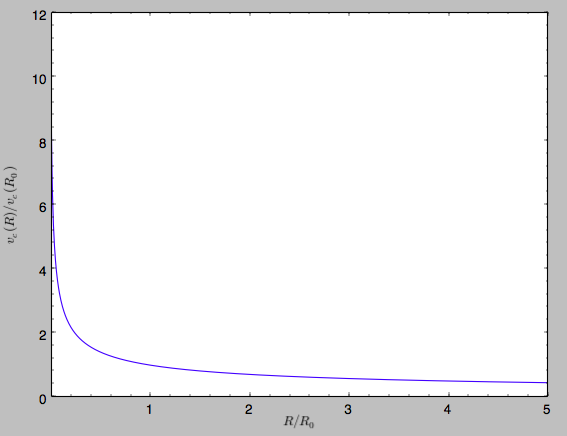Because evaluating the potential and forces of a snapshot is computationally expensive, most useful applications of frozen N-body potentials employ interpolated versions of the snapshot potential. These can be setup in galpy using an InterpSnapshotRZPotential class that is a subclass of the interpRZPotential described above and that can be used in the same manner. To illustrate its use we will make use of one of pynbody’s example snapshots, g15784. This snapshot is used here to illustrate pynbody’s use. Please follow the instructions there on how to download this snapshot.

Once you have downloaded the pynbody testdata, we can load this snapshot using

>>> s = pynbody.load('testdata/g15784.lr.01024.gz')


(please adjust the path according to where you downloaded the pynbody testdata). We get the main galaxy in this snapshot, center the simulation on it, and align the galaxy face-on using

>>> h = s.halos()
>>> h1 = h
>>> pynbody.analysis.halo.center(h1,mode='hyb')
>>> pynbody.analysis.angmom.faceon(h1, cen=(0,0,0),mode='ssc')


we also convert the simulation to physical units, but set G=1 by doing the following

>>> s.physical_units()
>>> from galpy.util.bovy_conversion import _G
>>> g= pynbody.array.SimArray(_G/1000.)
>>> g.units= 'kpc Msol**-1 km**2 s**-2 G**-1'
>>> s._arrays['mass']= s._arrays['mass']*g


We can now load an interpolated version of this snapshot’s potential into galpy using

>>> from galpy.potential import InterpSnapshotRZPotential
>>> spi= InterpSnapshotRZPotential(h1,rgrid=(numpy.log(0.01),numpy.log(20.),101),logR=True,zgrid=(0.,10.,101),interpPot=True,zsym=True)


where we further assume that the potential is symmetric around the mid-plane (z=0). This instantiation will take about ten to fiteen minutes. This potential instance has physical units (and thus the rgrid= and zgrid= inputs are given in kpc if the simulation’s distance unit is kpc). For example, if we ask for the rotation curve, we get the following:

>>> spi.plotRotcurve(Rrange=[0.01,19.9],xlabel=r'$R\,(\mathrm{kpc})$',ylabel=r'$v_c(R)\,(\mathrm{km\,s}^{-1})$')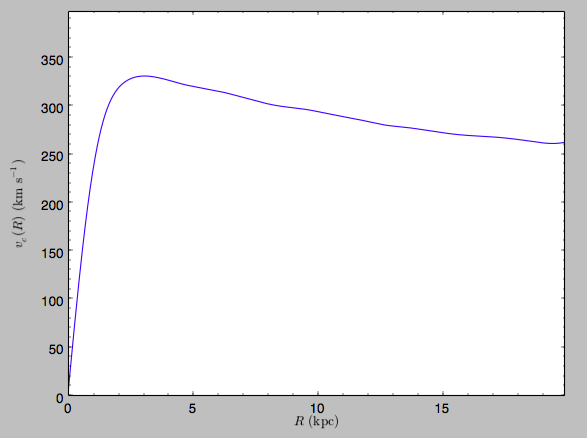This can be compared to the rotation curve calculated by pynbody, see here.

Because galpy works best in a system of natural units as explained in Units in galpy, we will convert this instance to natural units using the circular velocity at R=10 kpc, which is

>>> spi.vcirc(10.)
# 294.62723076942245


To convert to natural units we do

>>> spi.normalize(R0=10.)


We can then again plot the rotation curve, keeping in mind that the distance unit is now $$R_0$$

>>> spi.plotRotcurve(Rrange=[0.01,1.99])


which gives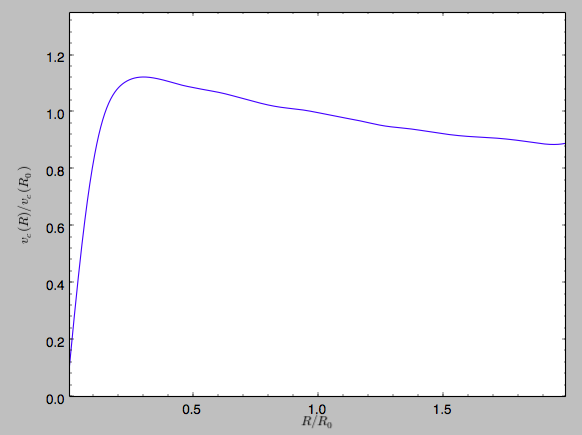in particular

>>> spi.vcirc(1.)
# 1.0000000000000002


We can also plot the potential

>>> spi.plot(rmin=0.01,rmax=1.9,nrs=51,zmin=-0.99,zmax=0.99,nzs=51)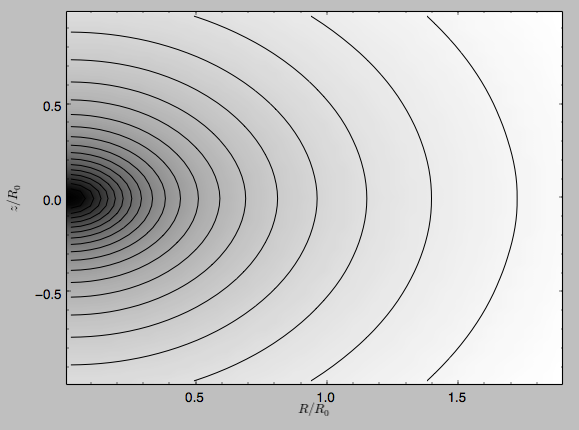Clearly, this simulation’s potential is quite spherical, which is confirmed by looking at the flattening

>>> spi.flattening(1.,0.1)
# 0.86675711023391921
>>> spi.flattening(1.5,0.1)
# 0.94442750306256895


The epicyle and vertical frequencies can also be interpolated by setting the interpepifreq=True or interpverticalfreq=True keywords when instantiating the InterpSnapshotRZPotential object.

## Conversion to NEMO potentials¶

NEMO is a set of tools for studying stellar dynamics. Some of its functionality overlaps with that of galpy, but many of its programs are very complementary to galpy. In particular, it has the ability to perform N-body simulations with a variety of poisson solvers, which is currently not supported by galpy (and likely will never be directly supported). To encourage interaction between galpy and NEMO it is quite useful to be able to convert potentials between these two frameworks, which is not completely trivial. In particular, NEMO contains Walter Dehnen’s fast collisionless gyrfalcON code (see 2000ApJ…536L..39D and 2002JCoPh.179…27D) and the discussion here focuses on how to run N-body simulations using external potentials defined in galpy.

Some galpy potential instances support the functions nemo_accname and nemo_accpars that return the name of the NEMO potential corresponding to this galpy Potential and its parameters in NEMO units. These functions assume that you use NEMO with WD_units, that is, positions are specified in kpc, velocities in kpc/Gyr, times in Gyr, and G=1. For the Miyamoto-Nagai potential above, you can get its name in the NEMO framework as

>>> mp.nemo_accname()
# 'MiyamotoNagai'


and its parameters as

>>> mp.nemo_accpars(220.,8.)
# '0,592617.11132,4.0,0.3'


assuming that we scale velocities by vo=220 km/s and positions by ro=8 kpc in galpy. These two strings can then be given to the gyrfalcON accname= and accpars= keywords.

We can do the same for lists of potentials. For example, for MWPotential2014 we do

>>> from galpy.potential import nemo_accname, nemo_accpars
>>> nemo_accname(MWPotential2014)
# 'PowSphwCut+MiyamotoNagai+NFW'
>>> nemo_accpars(MWPotential2014,220.,8.)
# '0,1001.79126907,1.8,1.9#0,306770.418682,3.0,0.28#0,16.0,162.958241887'


Therefore, these are the accname= and accpars= that one needs to provide to gyrfalcON to run a simulation in MWPotential2014.

Note that the NEMO potential PowSphwCut is not a standard NEMO potential. This potential can be found in the nemo/ directory of the galpy source code; this directory also contains a Makefile that can be used to compile the extra NEMO potential and install it in the correct NEMO directory (this requires one to have NEMO running, i.e., having sourced nemo_start).

You can use the PowSphwCut.cc file in the nemo/ directory as a template for adding additional potentials in galpy to the NEMO framework. To figure out how to convert the normalized galpy potential to an amplitude when scaling to physical coordinates (like kpc and kpc/Gyr), one needs to look at the scaling of the radial force with R. For example, from the definition of MiyamotoNagaiPotential, we see that the radial force scales as $$R^{-2}$$. For a general scaling $$R^{-\alpha}$$, the amplitude will scale as $$V_0^2\,R_0^{\alpha-1}$$ with the velocity $$V_0$$ and position $$R_0$$ of the v=1 at R=1 normalization. Therefore, for the MiyamotoNagaiPotential, the physical amplitude scales as $$V_0^2\,R_0$$. For the LogarithmicHaloPotential, the radial force scales as $$R^{-1}$$, so the amplitude scales as $$V_0^2$$.

Currently, only the MiyamotoNagaiPotential, NFWPotential, PowerSphericalPotentialwCutoff, HernquistPotential, PlummerPotential, MN3ExponentialDiskPotential, and the LogarithmicHaloPotential have this NEMO support. Combinations of all but the LogarithmicHaloPotential are allowed in general (e.g., MWPotential2014); they can also be combined with spherical LogarithmicHaloPotentials. Because of the definition of the logarithmic potential in NEMO, it cannot be flattened in z, so to use a flattened logarithmic potential, one has to flip y and z between galpy and NEMO (one can flatten in y).

## NEW in v1.5: Conversion to AMUSE potentials¶

AMUSE is a Python software framework for astrophysical simulations, in which existing codes from different domains, such as stellar dynamics, stellar evolution, hydrodynamics and radiative transfer can be easily coupled. AMUSE allows you to run N-body simulations that include a wide range of physics (gravity, stellar evolution, hydrodynamics, radiative transfer) with a large variety of numerical codes (collisionless, collisional, etc.).

The galpy.potential.to_amuse function allows you to create an AMUSE representation of any galpy potential. This is useful, for instance, if you want to run a simulation of a stellar cluster in an external gravitational field, because galpy has wide support for representing external gravitational fields. Creating the AMUSE representation is as simple as (for MWPotential2014):

>>> from galpy.potential import to_amuse, MWPotential2014
>>> mwp_amuse= to_amuse(MWPotential2014)
>>> print(mwp_amuse)
# <galpy.potential.amuse.galpy_profile object at 0x7f6b366d13c8>


Schematically, this potential can then be used in AMUSE as

>>> gravity = bridge.Bridge(use_threading=False)


where cluster_code is a code to perform the N-body integration of a system (e.g., a BHTree in AMUSE). A fuller example is given below.

AMUSE uses physical units when interacting with the galpy potential and it is therefore necessary to make sure that the correct physical units are used. The to_amuse function takes the galpy unit conversion parameters ro= and vo= as keyword parameters to perform the conversion between internal galpy units and physical units; if these are not explicitly set, to_amuse attempts to set them automatically using the potential that you input using the galpy.util.bovy_conversion.get_physical function.

Another difference between galpy and AMUSE is that in AMUSE integration times can only be positive and they have to increase in time. to_amuse takes as input the t= and tgalpy= keywords that specify (a) the initial time in AMUSE and (b) the initial time in galpy that this time corresponds to. Typically these will be the same (and equal to zero), but if you want to run a simulation where the initial time in galpy is negative it is useful to give them different values. The time inputs can be either given in galpy internal units or using AMUSE’s units. Similarly, to integrate backwards in time in AMUSE, to_amuse has a keyword reverse= (default: False) that reverses the time direction given to the galpy potential; reverse=True does this (note that you also have to flip the velocities to actually go backwards).

A full example of setting up a Plummer-sphere cluster and evolving its N-body dynamics using an AMUSE BHTree in the external MWPotential2014 potential is:

>>> from amuse.lab import *
>>> from amuse.couple import bridge
>>> from amuse.datamodel import Particles
>>> from galpy.potential import to_amuse, MWPotential2014
>>> from galpy.util import bovy_plot
>>>
>>> # Convert galpy MWPotential2014 to AMUSE representation
>>> mwp_amuse= to_amuse(MWPotential2014)
>>>
>>> # Set initial cluster parameters
>>> N= 1000
>>> Mcluster= 1000. | units.MSun
>>> Rcluster= 10. | units.parsec
>>> Rinit= [10.,0.,0.] | units.kpc
>>> Vinit= [0.,220.,0.] | units.km/units.s
>>> # Setup star cluster simulation
>>> tend= 100.0 | units.Myr
>>> dtout= 5.0 | units.Myr
>>> dt= 1.0 | units.Myr
>>>
>>> def setup_cluster(N,Mcluster,Rcluster,Rinit,Vinit):
>>>     converter= nbody_system.nbody_to_si(Mcluster,Rcluster)
>>>     stars= new_plummer_sphere(N,converter)
>>>     stars.x+= Rinit
>>>     stars.y+= Rinit
>>>     stars.z+= Rinit
>>>     stars.vx+= Vinit
>>>     stars.vy+= Vinit
>>>     stars.vz+= Vinit
>>>     return stars,converter
>>>
>>> # Setup cluster
>>> stars,converter= setup_cluster(N,Mcluster,Rcluster,Rinit,Vinit)
>>> cluster_code= BHTree(converter,number_of_workers=1) #Change number of workers depending no. of CPUs
>>> cluster_code.parameters.epsilon_squared= (3. | units.parsec)**2
>>> cluster_code.parameters.opening_angle= 0.6
>>> cluster_code.parameters.timestep= dt
>>>
>>> # Setup channels between stars particle dataset and the cluster code
>>> channel_from_stars_to_cluster_code= stars.new_channel_to(cluster_code.particles,
>>>                                        attributes=["mass", "x", "y", "z", "vx", "vy", "vz"])
>>> channel_from_cluster_code_to_stars= cluster_code.particles.new_channel_to(stars,
>>>                                        attributes=["mass", "x", "y", "z", "vx", "vy", "vz"])
>>>
>>> # Setup gravity bridge
>>> # Stars in cluster_code depend on gravity from external potential mwp_amuse (i.e., MWPotential2014)
>>> # External potential mwp_amuse still needs to be added to system so it evolves with time
>>> # Set how often to update external potential
>>> gravity.timestep= cluster_code.parameters.timestep/2.
>>> # Evolve
>>> time= 0.0 | tend.unit
>>> while time<tend:
>>>     gravity.evolve_model(time+dt)
>>>     # If you want to output or analyze the simulation, you need to copy
>>>     # stars from cluster_code
>>>     #channel_from_cluster_code_to_stars.copy()
>>>
>>>     # If you edited the stars particle set, for example to remove stars from the
>>>     # array because they have been kicked far from the cluster, you need to
>>>     # copy the array back to cluster_code:
>>>     #channel_from_stars_to_cluster_code.copy()
>>>
>>>     # Update time
>>>     time= gravity.model_time
>>>
>>> channel_from_cluster_code_to_stars.copy()
>>> gravity.stop()
>>>
>>> bovy_plot.bovy_plot(stars.x.value_in(units.kpc),stars.y.value_in(units.kpc),'.',
>>>                     xlabel=r'$X\,(\mathrm{kpc})$',ylabel=r'$Y\,(\mathrm{kpc})$')


After about 30 seconds, you should get a plot like the following, which shows a cluster in the first stages of disruption: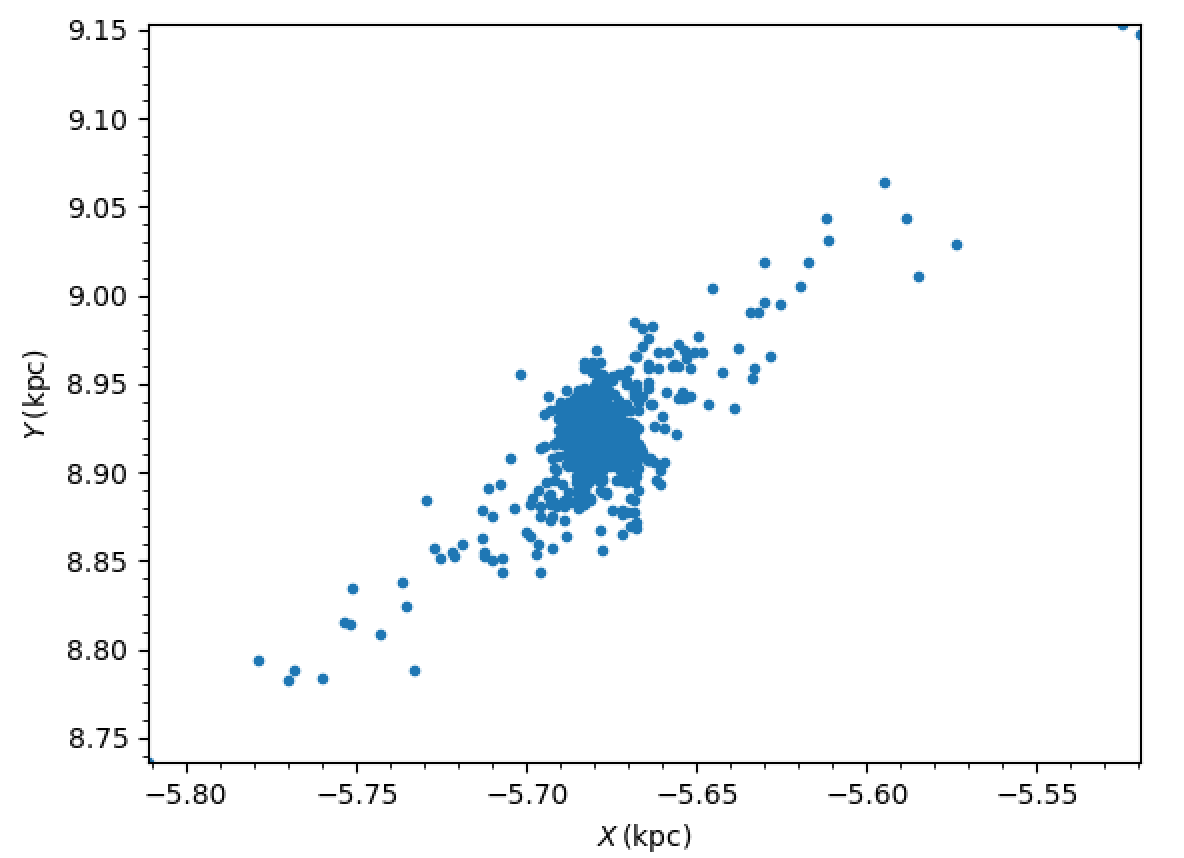## Dissipative forces¶

While almost all of the forces that you can use in galpy derive from a potential (that is, the force is the gradient of a scalar function, the potential, meaning that the forces are conservative), galpy also supports dissipative forces. Dissipative forces all inherit from the DissipativeForce class and they are required to take the velocity v=[vR,vT,vZ] in cylindrical coordinates as an argument to the force in addition to the standard (R,z,phi=0,t=0). The set of functions evaluateXforces (with X=R,z,r,phi,etc.) will evaluate the force due to Potential instances, DissipativeForce instances, or lists of combinations of these two.

Currently, the only dissipative force implemented in galpy is ChandrasekharDynamicalFrictionForce, an implementation of the classic Chandrasekhar dynamical-friction formula, with recent tweaks to better represent the results from N-body simulations.

Note that there is currently no support for implementing dissipative forces in C. Thus, only Python-based integration methods are available for any dissipative forces.

Warning

Dissipative forces can currently only be used for 3D orbits in galpy. The code should throw an error when they are used for 2D orbits.

## Adding potentials to the galpy framework¶

Potentials in galpy can be used in many places such as orbit integration, distribution functions, or the calculation of action-angle variables, and in most cases any instance of a potential class that inherits from the general Potential class (or a list of such instances) can be given. For example, all orbit integration routines work with any list of instances of the general Potential class. Adding new potentials to galpy therefore allows them to be used everywhere in galpy where general Potential instances can be used. Adding a new class of potentials to galpy consists of the following series of steps (for steps to add a new wrapper potential, also see the next section):

1. Implement the new potential in a class that inherits from galpy.potential.Potential. The new class should have an __init__ method that sets up the necessary parameters for the class. An amplitude parameter amp= and two units parameters ro= and vo= should be taken as an argument for this class and before performing any other setup, the galpy.potential.Potential.__init__(self,amp=amp,ro=ro,vo=vo,amp_units=) method should be called to setup the amplitude and the system of units; the amp_units= keyword specifies the physical units of the amplitude parameter (e.g., amp_units='velocity2' when the units of the amplitude are velocity-squared) To add support for normalizing the potential to standard galpy units, one can call the galpy.potential.Potential.normalize function at the end of the __init__ function.

The new potential class should implement some of the following functions:

• _evaluate(self,R,z,phi=0,t=0) which evaluates the potential itself (without the amp factor, which is added in the __call__ method of the general Potential class).
• _Rforce(self,R,z,phi=0.,t=0.) which evaluates the radial force in cylindrical coordinates (-d potential / d R).
• _zforce(self,R,z,phi=0.,t=0.) which evaluates the vertical force in cylindrical coordinates (-d potential / d z).
• _R2deriv(self,R,z,phi=0.,t=0.) which evaluates the second (cylindrical) radial derivative of the potential (d^2 potential / d R^2).
• _z2deriv(self,R,z,phi=0.,t=0.) which evaluates the second (cylindrical) vertical derivative of the potential (d^2 potential / d z^2).
• _Rzderiv(self,R,z,phi=0.,t=0.) which evaluates the mixed (cylindrical) radial and vertical derivative of the potential (d^2 potential / d R d z).
• _dens(self,R,z,phi=0.,t=0.) which evaluates the density. If not given, the density is computed using the Poisson equation from the first and second derivatives of the potential (if all are implemented).
• _mass(self,R,z=0.,t=0.) which evaluates the mass. For spherical potentials this should give the mass enclosed within the spherical radius; for axisymmetric potentials this should return the mass up to R and between -Z and Z. If not given, the mass is computed by integrating the density (if it is implemented or can be calculated from the Poisson equation).
• _phiforce(self,R,z,phi=0.,t=0.): the azimuthal force in cylindrical coordinates (assumed zero if not implemented).
• _phi2deriv(self,R,z,phi=0.,t=0.): the second azimuthal derivative of the potential in cylindrical coordinates (d^2 potential / d phi^2; assumed zero if not given).
• _Rphideriv(self,R,z,phi=0.,t=0.): the mixed radial and azimuthal derivative of the potential in cylindrical coordinates (d^2 potential / d R d phi; assumed zero if not given).
• OmegaP(self): returns the pattern speed for potentials with a pattern speed (used to compute the Jacobi integral for orbits).

If you want to be able to calculate the concentration for a potential, you also have to set self._scale to a scale parameter for your potential.

The code for galpy.potential.MiyamotoNagaiPotential gives a good template to follow for 3D axisymmetric potentials. Similarly, the code for galpy.potential.CosmphiDiskPotential provides a good template for 2D, non-axisymmetric potentials.

During development or if some of the forces or second derivatives are too tedious to implement, it is possible to numerically compute any non-implemented forces and second derivatives by inheriting from the NumericalPotentialDerivativesMixin class. Thus, a functioning potential can be implemented by simply implementing the _evaluate function and adding all forces and second derivatives using the NumericalPotentialDerivativesMixin.

After this step, the new potential will work in any part of galpy that uses pure python potentials. To get the potential to work with the C implementations of orbit integration or action-angle calculations, the potential also has to be implemented in C and the potential has to be passed from python to C.

The __init__ method should be written in such a way that a relevant object can be initialized using Classname() (i.e., there have to be reasonable defaults given for all parameters, including the amplitude); doing this allows the nose tests for potentials to automatically check that your Potential’s potential function, force functions, second derivatives, and density (through the Poisson equation) are correctly implemented (if they are implemented). The continuous-integration platform that builds the galpy codebase upon code pushes will then automatically test all of this, streamlining push requests of new potentials.

A few atrributes need to be set depending on the potential: hasC=True for potentials for which the forces and potential are implemented in C (see below); self.hasC_dxdv=True for potentials for which the (planar) second derivatives are implemented in C; self.isNonAxi=True for non-axisymmetric potentials.

1. To add a C implementation of the potential, implement it in a .c file under potential/potential_c_ext. Look at potential/potential_c_ext/LogarithmicHaloPotential.c for the right format for 3D, axisymmetric potentials, or at potential/potential_c_ext/LopsidedDiskPotential.c for 2D, non-axisymmetric potentials.

For orbit integration, the functions such as:

• double LogarithmicHaloPotentialRforce(double R,double Z, double phi,double t,struct potentialArg * potentialArgs)
• double LogarithmicHaloPotentialzforce(double R,double Z, double phi,double t,struct potentialArg * potentialArgs)

are most important. For some of the action-angle calculations

• double LogarithmicHaloPotentialEval(double R,double Z, double phi,double t,struct potentialArg * potentialArgs)

is most important (i.e., for those algorithms that evaluate the potential). The arguments of the potential are passed in a potentialArgs structure that contains args, which are the arguments that should be unpacked. Again, looking at some example code will make this clear. The potentialArgs structure is defined in potential/potential_c_ext/galpy_potentials.h.

3. Add the potential’s function declarations to potential/potential_c_ext/galpy_potentials.h

4. (4. and 5. for planar orbit integration) Edit the code under orbit/orbit_c_ext/integratePlanarOrbit.c to set up your new potential (in the parse_leapFuncArgs function).

5. Edit the code in orbit/integratePlanarOrbit.py to set up your new potential (in the _parse_pot function).

6. Edit the code under orbit/orbit_c_ext/integrateFullOrbit.c to set up your new potential (in the parse_leapFuncArgs_Full function).

7. Edit the code in orbit/integrateFullOrbit.py to set up your new potential (in the _parse_pot function).

8. (for using the actionAngleStaeckel methods in C) Edit the code in actionAngle/actionAngle_c_ext/actionAngle.c to parse the new potential (in the parse_actionAngleArgs function).

9. Finally, add self.hasC= True to the initialization of the potential in question (after the initialization of the super class, or otherwise it will be undone). If you have implemented the necessary second derivatives for integrating phase-space volumes, also add self.hasC_dxdv=True.

After following the relevant steps, the new potential class can be used in any galpy context in which C is used to speed up computations.

## Adding wrapper potentials to the galpy framework¶

Wrappers all inherit from the general WrapperPotential or planarWrapperPotential classes (which themselves inherit from the Potential and planarPotential classes and therefore all wrappers are Potentials or planarPotentials). Depending on the complexity of the wrapper, wrappers can be implemented much more economically in Python than new Potential instances as described above.

To add a Python implementation of a new wrapper, classes need to inherit from parentWrapperPotential, take the potentials to be wrapped as a pot= (a Potential, planarPotential, or a list thereof; automatically assigned to self._pot) input to __init__, and implement the _wrap(self,attribute,*args,**kwargs) function. This function modifies the Potential functions _evaluate, _Rforce, etc. (all of those listed above), with attribute the function that is being modified. Inheriting from parentWrapperPotential gives the class access to the self._wrap_pot_func(attribute) function which returns the relevant function for each attribute. For example, self._wrap_pot_func('_evaluate') returns the evaluatePotentials function that can then be called as self._wrap_pot_func('_evaluate')(self._pot,R,Z,phi=phi,t=t) to evaluate the potentials being wrapped. By making use of self._wrap_pot_func, wrapper potentials can be implemented in just a few lines. Your __init__ function should only initialize things in your wrapper; there is no need to manually assign self._pot or to call the superclass’ __init__ (all automatically done for you!).

To correctly work with both 3D and 2D potentials, inputs to _wrap need to be specified as *args,**kwargs: grab the values you need for R,z,phi,t from these as R=args, z=0 if len(args) == 1 else args, phi=kwargs.get('phi',0.), t=kwargs.get('t',0.), where the complicated expression for z is to correctly deal with both 3D and 2D potentials (of course, if your wrapper depends on z, it probably doesn’t make much sense to apply it to a 2D planarPotential; you could check the dimensionality of self._pot in your wrapper’s __init__ function with from galpy.potential.Potential._dim and raise an error if it is not 3 in this case). Wrapping a 2D potential automatically results in a wrapper that is a subclass of planarPotential rather than Potential; this is done by the setup in parentWrapperPotential and hidden from the user. For wrappers of planar Potentials, self._wrap_pot_func(attribute) will return the evaluateplanarPotentials etc. functions instead, but this is again hidden from the user if you implement the _wrap function as explained above.

As an example, for the DehnenSmoothWrapperPotential, the _wrap function is

def _wrap(self,attribute,*args,**kwargs):
return self._smooth(kwargs.get('t',0.))\
*self._wrap_pot_func(attribute)(self._pot,*args,**kwargs)


where smooth(t) returns the smoothing function of the amplitude. When any of the basic Potential functions are called (_evaluate, _Rforce, etc.), _wrap gets called by the superclass WrapperPotential, and the _wrap function returns the corresponding function for the wrapped potentials with the amplitude modified by smooth(t). Therefore, one does not need to implement each of the _evaluate, _Rforce, etc. functions like for regular potential. The rest of the DehnenSmoothWrapperPotential is essentially (slightly simplified in non-crucial aspects)

def __init__(self,amp=1.,pot=None,tform=-4.,tsteady=None,ro=None,vo=None):
# Note: (i) don't assign self._pot and (ii) don't run super.__init__
self._tform= tform
else:
self.hasC= True
self.hasC_dxdv= True

def _smooth(self,t):
#Calculate relevant time
if t < self._tform:
smooth= 0.
deltat= t-self._tform
smooth= (3./16.*xi**5.-5./8*xi**3.+15./16.*xi+.5)
else: #bar is fully on
smooth= 1.
return smooth


The source code for DehnenSmoothWrapperPotential potential may act as a guide to implementing new wrappers.

C implementations of potential wrappers can also be added in a similar way as C implementations of regular potentials (all of the steps listed in the previous section for adding a potential to C need to be followed). All of the necessary functions (...Rforce, ...zforce, ..phiforce, etc.) need to be implemented separately, but by including galpy_potentials.h calling the relevant functions of the wrapped potentials is easy. Look at DehnenSmoothWrapperPotential.c for an example that can be straightforwardly edited for other wrappers.

The glue between Python and C for wrapper potentials needs to glue both the wrapper and the wrapped potentials. This can be easily achieved by recursively calling the _parse_pot glue functions in Python (see the previous section; this needs to be done separately for each potential currently) and the parse_leapFuncArgs and parse_leapFuncArgs_Full functions in C (done automatically for all wrappers). Again, following the example of DehnenSmoothWrapperPotential.py should allow for a straightforward implementation of the glue for any new wrappers. Wrapper potentials should be given negative potential types in the glue to distinguish them from regular potentials.

## Adding dissipative forces to the galpy framework¶

Dissipative forces are implemented in much the same way as forces that derive from potentials. Rather than inheriting from galpy.potential.Potential, dissipative forces inherit from galpy.potential.DissipativeForce. The procedure for implementing a new class of dissipative force is therefore very similar to that for implementing a new potential. The main differences are that (a) you only need to implement the forces and (b) the forces are required to take an extra keyword argument v= that gives the velocity in cylindrical coordinates (because dissipative forces will in general depend on the current velocity). Thus, the steps are:

1. Implement the new dissipative force in a class that inherits from galpy.potential.DissipativeForce. The new class should have an __init__ method that sets up the necessary parameters for the class. An amplitude parameter amp= and two units parameters ro= and vo= should be taken as an argument for this class and before performing any other setup, the galpy.potential.DissipativeForce.__init__(self,amp=amp,ro=ro,vo=vo,amp_units=) method should be called to setup the amplitude and the system of units; the amp_units= keyword specifies the physical units of the amplitude parameter (e.g., amp_units='mass' when the units of the amplitude are mass)

The new dissipative-force class should implement the following functions:

• _Rforce(self,R,z,phi=0.,t=0.,v=None) which evaluates the radial force in cylindrical coordinates
• _phiforce(self,R,z,phi=0.,t=0.,v=None) which evaluates the azimuthal force in cylindrical coordinates
• _zforce(self,R,z,phi=0.,t=0.,v=None) which evaluates the vertical force in cylindrical coordinates

The code for galpy.potential.ChandrasekharDynamicalFrictionForce gives a good template to follow.

2. That’s it, as for now there is no support for implementing a C version of dissipative forces.